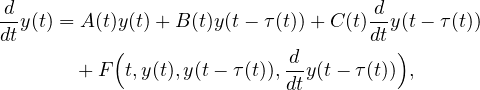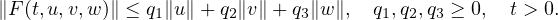Electron. J. Differential Equations, Vol. 2020 (2020), No. 20, pp. 1-12.

### Exponential stability of solutions to nonlinear time-varying delay systems of neutral type equations with periodic coefficients Author: Inessa I. Matveeva

Abstract:
We consider a class of nonlinear time-varying delay systems of neutral type differential equations with periodic coefficients in the linear terms,where A(t), B(t), C(t) are T-periodic matrices, andWe obtain conditions for the exponential stability of the zero solution and estimates for the exponential decay of the solutions at infinity.

Submitted April 30, 2019. Published February 14, 2020.
Math Subject Classifications: 34K20.
Key Words: Time-varying delay equation; neutral equation; periodic coefficient; exponential stability.

Show me the PDF file (324 KB), TEX file for this article.Inessa I. Matveeva Laboratory of Differential and Difference Equations Sobolev Institute of Mathematics 4, Acad. Koptyug avenue, Novosibirsk 630090, Russia email: matveeva@math.nsc.ru